Download Presentation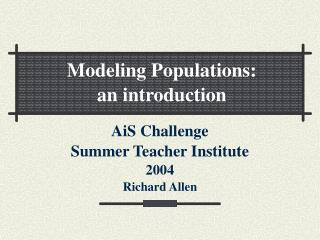AiS Challenge Summer Teacher Institute 2004 Richard Allen

# AiS Challenge Summer Teacher Institute 2004 Richard Allen - PowerPoint PPT Presentation

Modeling Populations: an introduction. AiS Challenge Summer Teacher Institute 2004 Richard Allen. Population Dynamics. Studies how populations change over timeI am the owner, or an agent authorized to act on behalf of the owner, of the copyrighted work described.
Download Presentation## AiS Challenge Summer Teacher Institute 2004 Richard Allen

An Image/Link below is provided (as is) to download presentation

Download Policy: Content on the Website is provided to you AS IS for your information and personal use and may not be sold / licensed / shared on other websites without getting consent from its author.While downloading, if for some reason you are not able to download a presentation, the publisher may have deleted the file from their server.

- - - - - - - - - - - - - - - - - - - - - - - - - - E N D - - - - - - - - - - - - - - - - - - - - - - - - - -
Presentation Transcript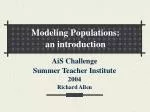Modeling Populations:

an introduction

AiS Challenge

Summer Teacher Institute

2004

Richard Allen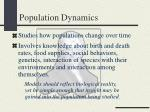Population Dynamics
• Studies how populations change over time
• Involves knowledge about birth and death rates, food supplies, social behaviors, genetics, interaction of species with their environments and interaction among themselves.

Models should reflect biological reality, yet be simple enough that insight maybegained into the population being studied.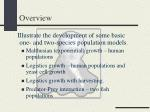Overview

Illustrate the development of some basic one- and two-species population models.

• Malthusian (exponential) growth – human populations
• Logistics growth – human populations and yeast cell growth
• Logistics growth with harvesting.
• Predator-Prey interaction – two fish populations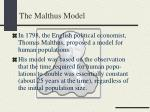The Malthus Model
• In 1798, the English political economist, Thomas Malthus, proposed a model for human populations.
• His model was based on the observation that the time required for human popu-lations to double was essentially constant (about 25 years at the time), regardless of the initial population size.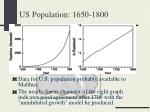US Population: 1650-1800
• Data for U.S. population probably available to Malthus.
• The nearly-linear character of the right graph indicates good agreement after 1700 with the "uninhibited growth" model he produced.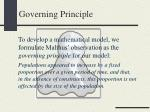Governing Principle

To develop a mathematical model, we formulate Malthus’ observation as the governing principle for our model:

Populations appeared to increase by a fixed proportion over a given period of time, and that, in the absence of constraints, this proportion is not affected by the size of the population.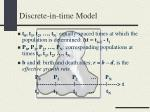Discrete-in-time Model
• t0, t1, t2, …, tN: equally-spaced times at which the population is determined: Δt = ti+1 - ti
• P0, P1, P2, …, PN: corresponding populations at times t0, t1, t2, …, tN
• b and d: birth and death rates; r = b – d, is the effective growth rate.

P0 P1 P2 … PN

|---------|---------|----------------|-----> t

t0 t1 t2 … tN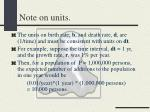Note on units.
• The units on birth rate, b, and death rate, d, are (1/time) and must be consistent with units on dt.
• For example, suppose the time interval, dt = 1 yr, and the growth rate, r, was 1% per year.
• Then, for a population of P = 1,000,000 persons, the expected number of additions to the population in one year would be

(0.01/year)*(1 year) * (1,000,000 persons) = 10,000 persons.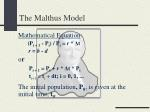The Malthus Model

Mathematical Equation:

(Pi + 1 - Pi) / Pi = r * Δt

r = b - d

or

Pi + 1 = Pi + r * Δt * Pi

ti+1 = ti + dt; i = 0, 1, ...

The initial population, P0, is given at the initial time, t0.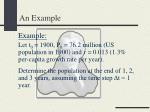An Example

Example:

Let t0 = 1900, P0 = 76.2 million (US population in 1900) and r = 0.013 (1.3% per-capita growth rate per year).

Determine the population at the end of 1, 2, and 3 years, assuming the time step Δt = 1 year.Example Calculation

P0 = 76.2; t0 = 1900; Δt = 1; r = 0.013

P1 = P0 + r* Δt*P0 = 76.2 + 0.013*1*76.2 = 77.3;

t1 = t0 + Δt = 1900 + 1 = 1901

P2 = P1 + r* Δt*P1 = 77.3 + 0.013*1*77.3 = 78.3;

t2 = t1 + Δt = 1901 + 1 = 1902

...

P2000 = 277.3 (284.5), t2000 = 2000US Population Prediction: Malthus

Malthus model prediction of the US population for the period 1900 - 2050, with initial data taken in 1900:

t0 = 1900; P0 = 76,200,000; r = 0.013

Actual US population given at 10-year intervals is also plotted for the period 1900-2000

Malthus PlotPseudo Code

INPUT:

t0 – initial time

P0 – initial population

Δt – length of time interval

N – number of time steps

r – population growth ratePseudo Code

OUTPUT

ti – ith time value

Pi – population at ti for i = 0, 1, …, N

ALGORITHM:

Set ti = t0

Set Pi = P0

Print ti, PiPseudo Code

for i = 1, 2, …, N

Set ti = ti + Δt

Set Pi = Pi + r* Δt * Pi

Print ti, Pi

end forLogistics Model

In 1838, Belgian mathematician Pierre Verhulst modified Malthus’ model to allow growth rate to depend on population:

r = [r0 * (1 – P/K)]

Pi+1 = Pi + [r0 * (1 - Pi/K)] *Δt* Pi

• r0 is maximum possible populationgrowth rate.
• Kis calledthe populationcarrying capacity.Logistics Model

Pi+1 = Pi + [r0* (1 - Pi/K)] *Δt* Pi

• ro controls not only population growth rate, but population decline rate (P > K); if reproduction is slow and mortality is fast, the logistic model will not work.
• Khas biological meaning for populations with strong interaction among individuals that control their reproduction: birds have territoriality, plants compete for space and light.Growth of Yeast Cells

Population of yeast cells grown under laboratory conditions: P0 = 10, K = 665, r0 = .54, Δt = 0.02US Population Prediction: Logistic

Logistic model prediction of the US population for the period 1900 – 2050, with initial data taken in 1900:

t0 = 1900; P0 = 76.2M; r0 = 0.017, K = 661.9

Actual US population given at 10-year inter-vals is also plotted for the period 1900-2000.

Logistic plotLogistics Growth with Harvesting

Harvesting populations, removing members from their environment, is a real-world phenomenon.

Assumptions:

• Per unit time, each member of the population has an equal chance of being harvested.
• In time period dt, expected number of harvests is f*dt*P where f is a harvesting intensity factor.Logistics Growth with Harvesting

The logistic model can easily by modified to include the effect of harvesting:

Pi+1 = Pi + r0* (1 – Pi / K) * Δt * Pi - f * Δt * Pi

or

Pi+1 = Pi + rh * (1 – Pi / Kh) *Δt * Pi

where

rh= r0 - f,Kh= [(r0 – f) / r0] * K

HarvestingA Predator-Prey Model: two competing fish populations

An early predator-prey model

• In the mid 1920’s the Italian biologist Umberto D’Ancona was studying the results of fishing on population variations of various species of fish that interact with each other.
• He came across data on the percentage-of-total-catch of several species of fish that were brought to different Mediterrian ports in the years that spanned World War ITwo Competing Fish Populations

Data for the port of Fiume, Italy for the years 1914 -1923: percentage-of-total-catch of predator fish (sharks, skates, rays, etc), not desirable as food fish.D’Amcona’ s Queries
• D’Amcona was puzzled by the large in-crease of predators during the war.
• He reasoned that this increase was due to the decrease in fishing during this period.
• Was this the case? What was the connec-tion between the intensity of fishing and the populations of food fish and predators?Two Competing Fish Populations
• The level of fishing and its effect on the two fish populations was also of concern to the fishing industry, since it would affect the way fishing was done.
• As any good scientist would do, D’Amcona con-tacted Vito Volterra, a local mathematician, to formulate a model for the growth of predators and their prey and the effect of fishing on the overall fish population.Strategy for Model Development

The model development is divided into three stages:

• In the absence of predators, prey population follows a logistics model and in the absence of prey, predators die out. Predator and prey do not interact with each other; no fishing allowed.
• The model is enhanced to allow for predator-prey interaction: predators consume prey
• Fishing is included in the modelOverall Model Assumptions

Simplifications

• Only two groups of fish:
• prey (food fish) and
• predators.
• No competing effects among predators
• No change in fish populations due to immigration into or emigration out of the physical region occupied by the fish.Model Variables

Notation

• ti - specific instances in time
• Fi - the prey population at time ti
• Si - the predator population at time ti
• rF - the growth rate of the prey in the absence of predators
• rS - the growth rate of the predators in the absence of prey
• K - the carrying capacity of preyStage 1: Basic Model

In the absence of predators, the fish population, F, is modeled by

Fi+1 = Fi + rF *Δt * Fi *(1 - Fi/K)

and in the absence of prey, the predator population, S, is modeled by

Si+1 = Si –rS *Δt *SiStage 2: Predator-Prey Interaction

a is the prey kill rate due to encounters with predators:

Fi+1 = Fi + rF*Δt*Fi*(1 - Fi/K) – a*Δt*Fi*Si

b is a parameter that converts prey-predator encounters to predator birth rate:

Si+1 = Si - rS*Δt*Si+ b*Δt*Fi*SiStage 3: Fishing

f is the effective fishing rate for both the predator and prey populations:

Fi+1 = Fi + rF*Δt*Fi*(1 - Fi/K) - a*Δt*Fi*Si- f*Δt*Fi

Si+1 = Si - rS*Δt*Si+ b*Δt*Fi*Si- f*Δt*SiModel Initial Conditions and Parameters

Plots for the input values:

t0 = 0.0 S0 = 100.0 F0 = 1000.0

dt = 0.02 N = 6000.0 f = 0.005

rS = 0.3rF = 0.5 a = 0.002

b = 0.0005 K = 4000.0 S0 = 100.0

Predator-Prey PlotsD’Ancona’s Question Answered (Model Solution)

A decrease in fishing, f, during WWI decreased the percentage of equilibrium prey population, F, and increased the percentage of equilibrium predator population, P.

f Prey Predators

0.1 800 (82.1%) 175 (17.9%)+

0.01 620 (74.9%) 208 (25.1%)

0.001 602 (74.0%) 212 (26.0%)

0.0001 600 (73.8%) 213 (26.2%)

+ (%) - percentage-of-total catch